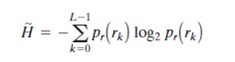Problem

# A 1024 × 1024 8-bit image with 5.3 bits/pixel entropy [computed from its histogram using E...

A 1024 × 1024 8-bit image with 5.3 bits/pixel entropy [computed from its histogram using Eq. (8.1-7)] is to be Huffman coded.

(a) What is the maximum compression that can be expected?

(b) Will it be obtained?

(c) If a greater level of lossless compression is required, what else can be done?(8.1-7)

#### Step-by-Step Solution

Solution 1

(a)

Number of bits required for representing the $$1024 \times 1024$$ image, $$n_{1}=8$$ bits.

Entropy of the $$1024 \times 1024$$ image, $$n_{2}=5.3$$ bits.

The maximum compression that can be expected is,

\begin{aligned} C &=\frac{n_{1}}{n_{2}} \\ &=\frac{8}{5.3} \\ &=1.51 \end{aligned}

(b)

The maximum compression of $$1.51$$ cannot be obtained as it requires the average length of the code words to be equal to entropy of the system, which is not possible.

Also, according to Shannon's first theorem (or noiseless coding theorem), a block of infinite symbols coded together would provide an average code word length equal to entropy.

$$\lim _{n \rightarrow \infty}\left[\frac{L_{a v g, n}}{n}\right]=H$$

Here,

$$L_{\text {avg }, n}$$ is the average number of code symbols required to represent all $$n$$-symbol groups.

However, the Huffman coding provides near optimal coding with code word length near to entropy and hence, a little less compression compared to $$1.51$$ can be achieved.

(c)

If a greater level of lossless compression is required, spatial or temporal redundancies in the image can be exploited. A simple way of obtaining more compression is to encode the adjacent pixel differences using Huffman coding. Huffman coding removes coding redundancy and yields the smallest possible number of code symbols per source symbol.